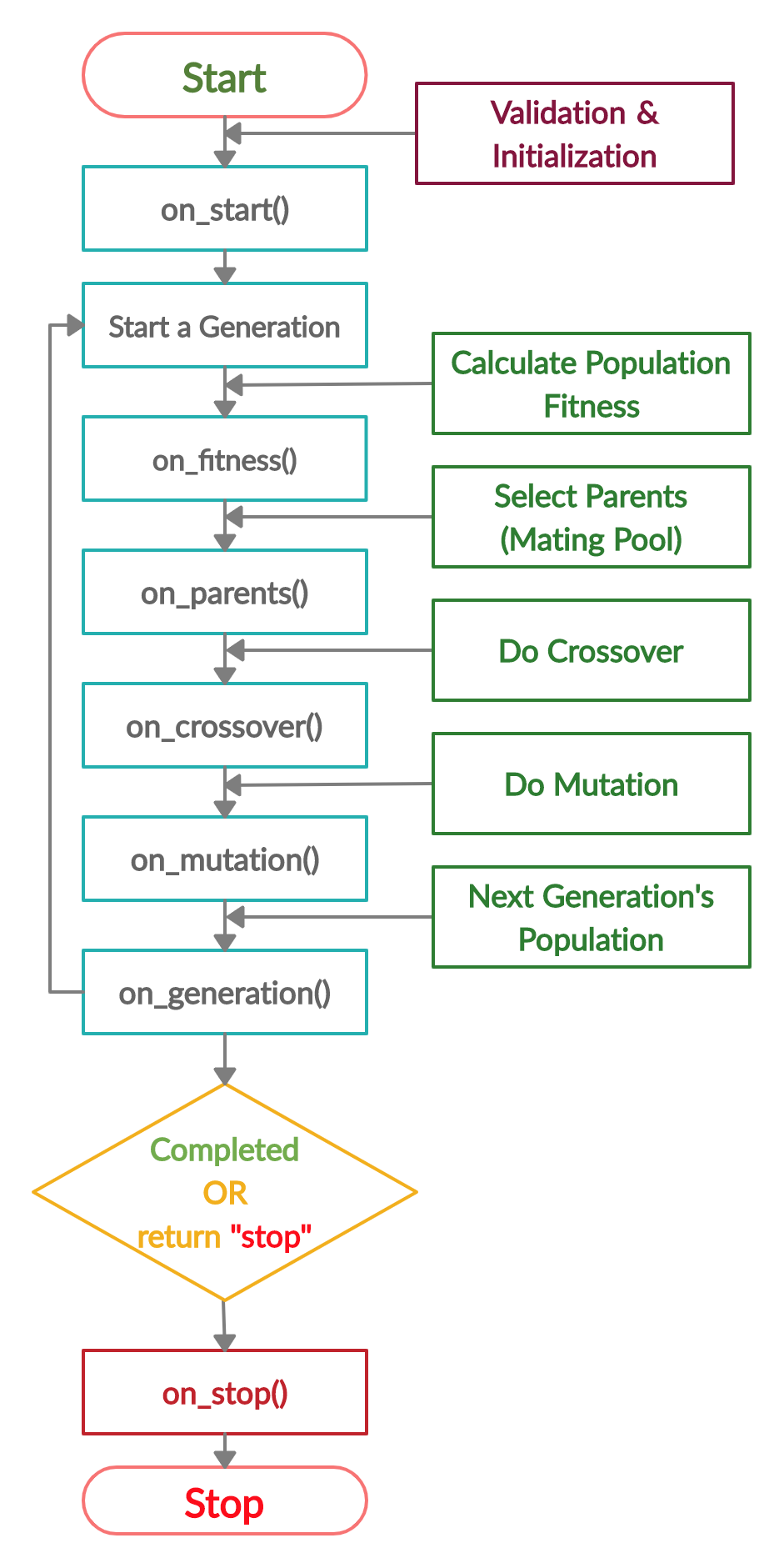## PyGAD: Genetic Algorithm in Python

This project is part of PyGAD which is an open-source Python 3 library for building the genetic algorithm and optimizing machine learning algorithms (Keras & PyTorch).

Check documentation of the GeneticAlgorithmPython project in the PyGAD's documentation: https://pygad.readthedocs.io/en/latest/README_pygad_ReadTheDocs.html

PyGAD supports different types of crossover, mutation, and parent selection. PyGAD allows different types of problems to be optimized using the genetic algorithm by customizing the fitness function.

The library is under active development and more features are added regularly. If you want a feature to be supported, please check the Contact Us section to send a request.

# Life Cycle of PyGAD

The next figure lists the different stages in the lifecycle of an instance of the `pygad.GA` class. Note that PyGAD stops when either all generations are completed or when the function passed to the `on_generation` parameter returns the string `stop`.The next code implements all the callback functions to trace the execution of the genetic algorithm. Each callback function prints its name.

``````import pygad
import numpy

function_inputs = [4,-2,3.5,5,-11,-4.7]
desired_output = 44

def fitness_func(solution, solution_idx):
output = numpy.sum(solution*function_inputs)
fitness = 1.0 / (numpy.abs(output - desired_output) + 0.000001)
return fitness

fitness_function = fitness_func

def on_start(ga_instance):
print("on_start()")

def on_fitness(ga_instance, population_fitness):
print("on_fitness()")

def on_parents(ga_instance, selected_parents):
print("on_parents()")

def on_crossover(ga_instance, offspring_crossover):
print("on_crossover()")

def on_mutation(ga_instance, offspring_mutation):
print("on_mutation()")

def on_generation(ga_instance):
print("on_generation()")

def on_stop(ga_instance, last_population_fitness):
print("on_stop()")

ga_instance = pygad.GA(num_generations=3,
num_parents_mating=5,
fitness_func=fitness_function,
sol_per_pop=10,
num_genes=len(function_inputs),
on_start=on_start,
on_fitness=on_fitness,
on_parents=on_parents,
on_crossover=on_crossover,
on_mutation=on_mutation,
on_generation=on_generation,
on_stop=on_stop)

ga_instance.run()
``````

Based on the used 3 generations as assigned to the `num_generations` argument, here is the output.

``````on_start()

on_fitness()
on_parents()
on_crossover()
on_mutation()
on_generation()

on_fitness()
on_parents()
on_crossover()
on_mutation()
on_generation()

on_fitness()
on_parents()
on_crossover()
on_mutation()
on_generation()

on_stop()
``````

# Example

Check the PyGAD's documentation for information about the implementation of this example.

``````import pygad
import numpy

"""
Given the following function:
y = f(w1:w6) = w1x1 + w2x2 + w3x3 + w4x4 + w5x5 + 6wx6
where (x1,x2,x3,x4,x5,x6)=(4,-2,3.5,5,-11,-4.7) and y=44
What are the best values for the 6 weights (w1 to w6)? We are going to use the genetic algorithm to optimize this function.
"""

function_inputs = [4,-2,3.5,5,-11,-4.7] # Function inputs.
desired_output = 44 # Function output.

def fitness_func(solution, solution_idx):
# Calculating the fitness value of each solution in the current population.
# The fitness function calulates the sum of products between each input and its corresponding weight.
output = numpy.sum(solution*function_inputs)
fitness = 1.0 / numpy.abs(output - desired_output)
return fitness

fitness_function = fitness_func

num_generations = 100 # Number of generations.
num_parents_mating = 7 # Number of solutions to be selected as parents in the mating pool.

# To prepare the initial population, there are 2 ways:
# 1) Prepare it yourself and pass it to the initial_population parameter. This way is useful when the user wants to start the genetic algorithm with a custom initial population.
# 2) Assign valid integer values to the sol_per_pop and num_genes parameters. If the initial_population parameter exists, then the sol_per_pop and num_genes parameters are useless.
sol_per_pop = 50 # Number of solutions in the population.
num_genes = len(function_inputs)

init_range_low = -2
init_range_high = 5

parent_selection_type = "sss" # Type of parent selection.
keep_parents = 7 # Number of parents to keep in the next population. -1 means keep all parents and 0 means keep nothing.

crossover_type = "single_point" # Type of the crossover operator.

# Parameters of the mutation operation.
mutation_type = "random" # Type of the mutation operator.
mutation_percent_genes = 10 # Percentage of genes to mutate. This parameter has no action if the parameter mutation_num_genes exists or when mutation_type is None.

last_fitness = 0
def callback_generation(ga_instance):
global last_fitness
print("Generation = {generation}".format(generation=ga_instance.generations_completed))
print("Fitness    = {fitness}".format(fitness=ga_instance.best_solution()))
print("Change     = {change}".format(change=ga_instance.best_solution() - last_fitness))
last_fitness = ga_instance.best_solution()

# Creating an instance of the GA class inside the ga module. Some parameters are initialized within the constructor.
ga_instance = pygad.GA(num_generations=num_generations,
num_parents_mating=num_parents_mating,
fitness_func=fitness_function,
sol_per_pop=sol_per_pop,
num_genes=num_genes,
init_range_low=init_range_low,
init_range_high=init_range_high,
parent_selection_type=parent_selection_type,
keep_parents=keep_parents,
crossover_type=crossover_type,
mutation_type=mutation_type,
mutation_percent_genes=mutation_percent_genes,
on_generation=callback_generation)

# Running the GA to optimize the parameters of the function.
ga_instance.run()

# After the generations complete, some plots are showed that summarize the how the outputs/fitenss values evolve over generations.
ga_instance.plot_result()

# Returning the details of the best solution.
solution, solution_fitness, solution_idx = ga_instance.best_solution()
print("Parameters of the best solution : {solution}".format(solution=solution))
print("Fitness value of the best solution = {solution_fitness}".format(solution_fitness=solution_fitness))
print("Index of the best solution : {solution_idx}".format(solution_idx=solution_idx))

prediction = numpy.sum(numpy.array(function_inputs)*solution)
print("Predicted output based on the best solution : {prediction}".format(prediction=prediction))

if ga_instance.best_solution_generation != -1:
print("Best fitness value reached after {best_solution_generation} generations.".format(best_solution_generation=ga_instance.best_solution_generation))

# Saving the GA instance.
filename = 'genetic' # The filename to which the instance is saved. The name is without extension.
ga_instance.save(filename=filename)

# Loading the saved GA instance.
loaded_ga_instance = pygad.load(filename=filename)
loaded_ga_instance.plot_result()
``````

## GitHub

https://github.com/ahmedfgad/GeneticAlgorithmPython